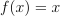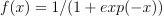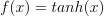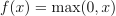# module `mlmodel.quantile_mlpregressor`#

## Short summary#

module `mlinsights.mlmodel.quantile_mlpregressor`

Implements a quantile non-linear regression.

source on GitHub

## Classes#

class

truncated documentation

`CustomizedMultilayerPerceptron`

Customized MLP Perceptron based on BaseMultilayerPerceptron. …

`QuantileMLPRegressor`

Quantile MLP Regression or neural networks regression trained with norm L1. This class inherits from sklearn.neural_networks.MLPRegressor. …

## Functions#

function

truncated documentation

`absolute_loss`

Computes the absolute loss for regression.

`float_sign`

Returns 1 if a > 0, otherwise -1

## Properties#

property

truncated documentation

`_repr_html_`

HTML representation of estimator. This is redundant with the logic of _repr_mimebundle_. The latter should …

`_repr_html_`

HTML representation of estimator. This is redundant with the logic of _repr_mimebundle_. The latter should …

## Methods#

method

truncated documentation

`__init__`

`__init__`

`_backprop`

Computes the MLP loss function and its corresponding derivatives with respect to each parameter: weights and bias …

`_backprop`

Computes the MLP loss function and its corresponding derivatives with respect to each parameter: weights and bias …

`_get_loss_function`

Returns the loss functions.

`_get_loss_function`

Returns the loss functions.

`_modify_loss_derivatives`

Modifies the loss derivatives.

`_modify_loss_derivatives`

Modifies the loss derivatives.

`_validate_input`

`predict`

Predicts using the multi-layer perceptron model.

`score`

Returns mean absolute error regression loss.

## Documentation#

Implements a quantile non-linear regression.

source on GitHub

class mlinsights.mlmodel.quantile_mlpregressor.CustomizedMultilayerPerceptron(hidden_layer_sizes, activation, solver, alpha, batch_size, learning_rate, learning_rate_init, power_t, max_iter, loss, shuffle, random_state, tol, verbose, warm_start, momentum, nesterovs_momentum, early_stopping, validation_fraction, beta_1, beta_2, epsilon, n_iter_no_change, max_fun)#

Bases: `BaseMultilayerPerceptron`

Customized MLP Perceptron based on BaseMultilayerPerceptron.

source on GitHub

__abstractmethods__ = frozenset({})#
__init__(hidden_layer_sizes, activation, solver, alpha, batch_size, learning_rate, learning_rate_init, power_t, max_iter, loss, shuffle, random_state, tol, verbose, warm_start, momentum, nesterovs_momentum, early_stopping, validation_fraction, beta_1, beta_2, epsilon, n_iter_no_change, max_fun)#
_abc_impl = <_abc._abc_data object>#

Computes the MLP loss function and its corresponding derivatives with respect to each parameter: weights and bias vectors.

Parameters:
• X – {array-like, sparse matrix}, shape (n_samples, n_features) The input data.

• y – array-like, shape (n_samples,) The target values.

• activations – list, length = n_layers - 1 The ith element of the list holds the values of the ith layer.

• deltas – list, length = n_layers - 1 The ith element of the list holds the difference between the activations of the i + 1 layer and the backpropagated error. More specifically, deltas are gradients of loss with respect to z in each layer, where z = wx + b is the value of a particular layer before passing through the activation function

• coef_grads – list, length = n_layers - 1 The ith element contains the amount of change used to update the coefficient parameters of the ith layer in an iteration.

• intercept_grads – list, length = n_layers - 1 The ith element contains the amount of change used to update the intercept parameters of the ith layer in an iteration.

Returns:

loss, float

Returns:

coef_grads, list, length = n_layers - 1

Returns:

intercept_grads, list, length = n_layers - 1

source on GitHub

_get_loss_function(loss_func_name)#

Returns the loss functions.

Parameters:

loss_func_name – loss function name, see sklearn.neural_networks.MLPRegressor

source on GitHub

_modify_loss_derivatives(last_deltas)#

Modifies the loss derivatives.

Parameters:

last_deltas – last deltas is the difference between the output and the expected output

Returns:

modified derivatives

source on GitHub

class mlinsights.mlmodel.quantile_mlpregressor.QuantileMLPRegressor(hidden_layer_sizes=(100,), activation='relu', solver='adam', alpha=0.0001, batch_size='auto', learning_rate='constant', learning_rate_init=0.001, power_t=0.5, max_iter=200, shuffle=True, random_state=None, tol=0.0001, verbose=False, warm_start=False, momentum=0.9, nesterovs_momentum=True, early_stopping=False, validation_fraction=0.1, beta_1=0.9, beta_2=0.999, epsilon=1e-08, n_iter_no_change=10, **kwargs)#

Quantile MLP Regression or neural networks regression trained with norm L1. This class inherits from sklearn.neural_networks.MLPRegressor. This model optimizes the absolute-loss using LBFGS or stochastic gradient descent. See `CustomizedMultilayerPerceptron` and `absolute_loss`.

Parameters:
• hidden_layer_sizes – tuple, length = n_layers - 2, default (100,) The ith element represents the number of neurons in the ith hidden layer.

• activation

{‘identity’, ‘logistic’, ‘tanh’, ‘relu’}, default ‘relu’ Activation function for the hidden layer. - ‘identity’, no-op activation, useful to implement linear bottleneck,

returns• ’logistic’, the logistic sigmoid function, returns.

• ’tanh’, the hyperbolic tan function, returns.

• ’relu’, the rectified linear unit function, returns.

• solver

`{'lbfgs', 'sgd', 'adam'}`, default ‘adam’ The solver for weight optimization. - ‘lbfgs’ is an optimizer in the family of quasi-Newton methods. - ‘sgd’ refers to stochastic gradient descent. - ‘adam’ refers to a stochastic gradient-based optimizer proposed by

Kingma, Diederik, and Jimmy Ba

Note: The default solver ‘adam’ works pretty well on relatively large datasets (with thousands of training samples or more) in terms of both training time and validation score. For small datasets, however, ‘lbfgs’ can converge faster and perform better.

• alpha – float, optional, default 0.0001 L2 penalty (regularization term) parameter.

• batch_size – int, optional, default ‘auto’ Size of minibatches for stochastic optimizers. If the solver is ‘lbfgs’, the classifier will not use minibatch. When set to “auto”, batch_size=min(200, n_samples)

• learning_rate

{‘constant’, ‘invscaling’, ‘adaptive’}, default ‘constant’ Learning rate schedule for weight updates. - ‘constant’ is a constant learning rate given by

’learning_rate_init’.

• ’invscaling’ gradually decreases the learning rate `learning_rate_` at each time step ‘t’ using an inverse scaling exponent of ‘power_t’. effective_learning_rate = learning_rate_init / pow(t, power_t)

• ’adaptive’ keeps the learning rate constant to ‘learning_rate_init’ as long as training loss keeps decreasing. Each time two consecutive epochs fail to decrease training loss by at least tol, or fail to increase validation score by at least tol if ‘early_stopping’ is on, the current learning rate is divided by 5.

Only used when solver=’sgd’.

• learning_rate_init – double, optional, default 0.001 The initial learning rate used. It controls the step-size in updating the weights. Only used when solver=’sgd’ or ‘adam’.

• power_t – double, optional, default 0.5 The exponent for inverse scaling learning rate. It is used in updating effective learning rate when the learning_rate is set to ‘invscaling’. Only used when solver=’sgd’.

• max_iter – int, optional, default 200 Maximum number of iterations. The solver iterates until convergence (determined by ‘tol’) or this number of iterations. For stochastic solvers (‘sgd’, ‘adam’), note that this determines the number of epochs (how many times each data point will be used), not the number of gradient steps.

• shuffle – bool, optional, default True Whether to shuffle samples in each iteration. Only used when solver=’sgd’ or ‘adam’.

• random_state – int, RandomState instance or None, optional, default None If int, random_state is the seed used by the random number generator; If RandomState instance, random_state is the random number generator; If None, the random number generator is the RandomState instance used by np.random.

• tol – float, optional, default 1e-4 Tolerance for the optimization. When the loss or score is not improving by at least `tol` for `n_iter_no_change` consecutive iterations, unless `learning_rate` is set to ‘adaptive’, convergence is considered to be reached and training stops.

• verbose – bool, optional, default False Whether to print progress messages to stdout.

• warm_start – bool, optional, default False When set to True, reuse the solution of the previous call to fit as initialization, otherwise, just erase the previous solution. See the Glossary.

• momentum – float, default 0.9 Momentum for gradient descent update. Should be between 0 and 1. Only used when solver=’sgd’.

• nesterovs_momentum – boolean, default True Whether to use Nesterov’s momentum. Only used when solver=’sgd’ and momentum > 0.

• early_stopping – bool, default False Whether to use early stopping to terminate training when validation score is not improving. If set to true, it will automatically set aside 10% of training data as validation and terminate training when validation score is not improving by at least `tol` for `n_iter_no_change` consecutive epochs. Only effective when solver=’sgd’ or ‘adam’

• validation_fraction – float, optional, default 0.1 The proportion of training data to set aside as validation set for early stopping. Must be between 0 and 1. Only used if early_stopping is True

• beta_1 – float, optional, default 0.9 Exponential decay rate for estimates of first moment vector in adam, should be in [0, 1). Only used when solver=’adam’

• beta_2 – float, optional, default 0.999 Exponential decay rate for estimates of second moment vector in adam, should be in [0, 1). Only used when solver=’adam’

• epsilon – float, optional, default 1e-8 Value for numerical stability in adam. Only used when solver=’adam’

• n_iter_no_change – int, optional, default 10 Maximum number of epochs to not meet `tol` improvement. Only effective when solver=’sgd’ or ‘adam’

Fitted attributes:

• loss_: float

The current loss computed with the loss function.

• coefs_: list, length n_layers - 1

The ith element in the list represents the weight matrix corresponding to layer i.

• intercepts_: list, length n_layers - 1

The ith element in the list represents the bias vector corresponding to layer i + 1.

• n_iter_: int,

The number of iterations the solver has ran.

• n_layers_: int

Number of layers.

• n_outputs_: int

Number of outputs.

• out_activation_: string

Name of the output activation function.

source on GitHub

source on GitHub

__abstractmethods__ = frozenset({})#
__init__(hidden_layer_sizes=(100,), activation='relu', solver='adam', alpha=0.0001, batch_size='auto', learning_rate='constant', learning_rate_init=0.001, power_t=0.5, max_iter=200, shuffle=True, random_state=None, tol=0.0001, verbose=False, warm_start=False, momentum=0.9, nesterovs_momentum=True, early_stopping=False, validation_fraction=0.1, beta_1=0.9, beta_2=0.999, epsilon=1e-08, n_iter_no_change=10, **kwargs)#

source on GitHub

_abc_impl = <_abc._abc_data object>#
_validate_input(X, y, incremental, reset=False)#
predict(X)#

Predicts using the multi-layer perceptron model.

Parameters:

X – {array-like, sparse matrix}, shape (n_samples, n_features) The input data.

Returns:

y : array-like, shape (n_samples, n_outputs) The predicted values.

source on GitHub

score(X, y, sample_weight=None)#

Returns mean absolute error regression loss.

Parameters:
• X – array-like, shape = (n_samples, n_features) Test samples.

• y – array-like, shape = (n_samples) or (n_samples, n_outputs) True values for X.

• sample_weight – array-like, shape = [n_samples], optional Sample weights.

Returns:

score, float mean absolute error regression loss

source on GitHub

mlinsights.mlmodel.quantile_mlpregressor.absolute_loss(y_true, y_pred)#

Computes the absolute loss for regression.

Parameters:
• y_true – array-like or label indicator matrix Ground truth (correct) values.

• y_pred – array-like or label indicator matrix Predicted values, as returned by a regression estimator.

Returns:

loss, float The degree to which the samples are correctly predicted.

source on GitHub

mlinsights.mlmodel.quantile_mlpregressor.float_sign(a)#

Returns 1 if a > 0, otherwise -1